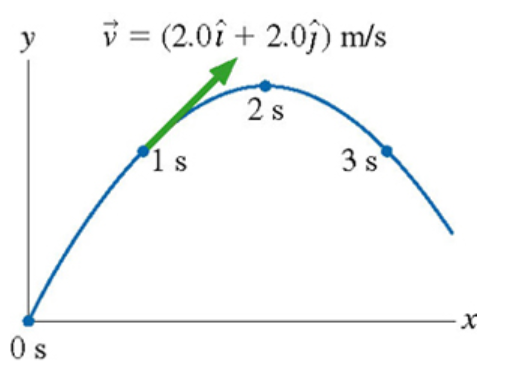# Problem: A physics student on Planet Exidor throws a ball, and it follows the parabolic trajectory shown in the figure. The ball's position is shown at 1s intervals until t=3s. At t=0s , the ball's velocity is v = (2.0 î + 4.0 ĵ) m/s.. Determine the ball's velocity at t = 3s

###### FREE Expert Solution

Uniform accelerated motion (UAM) equations:

v0 = (2.0 i + 4.0 j) m/s

90% (349 ratings)###### Problem Details

A physics student on Planet Exidor throws a ball, and it follows the parabolic trajectory shown in the figure. The ball's position is shown at 1s intervals until t=3s. At t=0s , the ball's velocity is v = (2.0 + 4.0 ) m/s..

Determine the ball's velocity at t = 3s